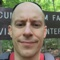What Is Flat Map?

January 10, 2019

This is a small program demonstrating the difference between the map() and flatMap() methods of the Java Stream class. I use map() extensively but rarely use flatMap(). That's because most of my algorithms operate on each item of the Stream one-at-a-time in a pipeline. flatMap() will produce more items by creating its own Stream.

This is a two-field class do demonstrate the methods.

class MyClass {

private final String fieldA;
private final String fieldB;

public MyClass(String fieldA, String fieldB) {
this.fieldA = fieldA;
this.fieldB = fieldB;
}

public String getFieldA() {
return fieldA;
}

public String getFieldB() {
return fieldB;
}
}

This next section of code will set up a List of test data. As each item proceeds through the Stream, map() converts the item in a one-to-one correspondence to a String.

List<MyClass> someData = new ArrayList<gt;();

someData.add( new MyClass( "c", "d") );
someData.add( new MyClass( "e", "f") );

System.out.println("Example #1 : Map MyClass objects to String");

someData
.stream()
.map( item -> String.format("%s %s", item.getFieldA(), item.getFieldB()) )
.forEach( s -> System.out.println(s) );

flatMap() returns a Stream. It could return a Stream of single items as with map(). In that case, there would still be a one-to-one correspondence between the input to flatMap() and it's output. Instead, this usage will produce two items for each input. The return value is a Stream rather than a Pair or other aggregation.

System.out.println();
System.out.println("Example #2 : Produce new Stream from MyClass objects" );

someData
.stream()
.flatMap( item -> Stream.of( item.getFieldA(), item.getFieldB() ) )
.forEach( a -> System.out.println(a) );

Running the program produces different numbers of lines for each test. In the case of map(), three lines are printed corresponding to each String produced from each element of input. For the flatMap() example, the number of lines printed is doubled. That's because flatMap() is taking each item and making a second two-item Stream. Hence, six lines are printed.